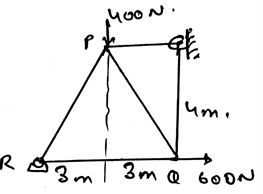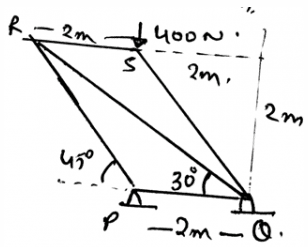Courses

# Test: Space Trusses

## 15 Questions MCQ Test Engineering Mechanics | Test: Space Trusses

Description
This mock test of Test: Space Trusses for Mechanical Engineering helps you for every Mechanical Engineering entrance exam. This contains 15 Multiple Choice Questions for Mechanical Engineering Test: Space Trusses (mcq) to study with solutions a complete question bank. The solved questions answers in this Test: Space Trusses quiz give you a good mix of easy questions and tough questions. Mechanical Engineering students definitely take this Test: Space Trusses exercise for a better result in the exam. You can find other Test: Space Trusses extra questions, long questions & short questions for Mechanical Engineering on EduRev as well by searching above.
QUESTION: 1

### __________ is a structure made of slender members which are joined together at their end points.

Solution:

The space truss is a structure made of slender members which are joined together at their end points. They can be of wooden or steel. But most probably they are made from stainless steel. As they need to support the loadings in various climates.

QUESTION: 2

### As the loading is acting in the three dimensions, that is in a multi planes. Thus the calculations involved in the trusses are in 3D.

Solution:

The loading is acting in a plane. Thus the calculations are done in 2D only. As the equations for 3D are different. Although the use of vectors can make our task easy but still 2D calculations are done for the trusses, as they are acting in a same plane.

QUESTION: 3

### Which of the following is correct for space trusses?

Solution:

The direction of the unknown is not known to us. To know the direction of the unknown force we take assumption of it. That is we assume that the particular direction might be the direction of the force and then we do the calculations accordingly.

QUESTION: 4

Find the force in the member RQ.Solution:

The direction of the unknown is not known to us. To know the direction of the unknown force we take assumption of it. That is we assume that the particular direction might be the direction of the force and then we do the calculations accordingly. And then we apply the equilibrium equations to the joints.

QUESTION: 5

As the loading in bridge different from the simple trusses the calculations involved in the bridges are all 2D calculations.

Solution:

The loading in the bridge is acting in a plane. Thus the calculations are done in 2D only. As the equations for 3D are different. Although the use of vectors can make our task easy but still 2D calculations are done for the trusses, as they are acting in a same plane. But the thing is that the loading in bridge and trusses are same.

QUESTION: 6

In case of bridge the load is transferred as:

Solution:

The bridge load is transmitted to the stringers first. It is then transmitted to the floor beams. The load from beams is then transmitted to the joints. Thus the transmission of the load of the bridge.

QUESTION: 7

To design the space trusses which of the following rules is followed?

Solution:

The set of rules which are used to design the trusses are having various rules. To them one is that the loads are applied at the joints. This is done with the neglecting of the weight of the trusses section.

QUESTION: 8

The rules which are used to design the space trusses are having various rules. Of them one is that the smooth pins are not used to join the members.

Solution:

The set of rules used to design the trusses are having various rules. Of them one is that the smooth pins are used to join the members. The joint are generally formed by welding the materials at the ends of the trusses. Which gives strength to the design.

QUESTION: 9

In a roof supporting space truss the load is transmitted as:

Solution:

The roof load is transmitted to the truss first. It is then transmitted to the joints. This transmission of the force or the load from trusses to the joints is achieved by the purlins. Thus the distribution of the load.

QUESTION: 10

The free body diagram of which part of the section of the space truss is made to make use of method of joints?

Solution:

The free body diagram of joints part of the section of the truss is made to make use of method of joints. Various equations of equilibrium are made to do the calculations. The net force acting on the vertical direction is made zero. Also horizontal direction forces are made to be zero.

QUESTION: 11

Find the force in the member RQ of the frame shown below.Solution:

The direction of the unknown is not known to us. To know the direction of the unknown force we take assumption of it. That is we assume that the particular direction might be the direction of the force and then we do the calculations accordingly. And then we apply the equilibrium equations to the joints.

QUESTION: 12

We use the method of joint to find the forces acting over the joints in the space trusses. In this we start from the joint having at least one known force and at the most two unknown forces.

Solution:

This makes the calculations easy. Also for the solving of any equation one requires equal number of variables as that of equal number of equation. So to do the calculations easily the above mentioned conditions are followed.

QUESTION: 13

As space trusses are used in bridges. When the bridges are extended over long routes or distance then:

Solution:

When the bridges are extended over long routes or distance then a rocker or a roller is used at the joints. This allows the bridge joints to move around. That is when the temperature is raised. The elongations and the contractions of the joints part are not much affected if the rollers and rockers are used.

QUESTION: 14

What is after taking the assumption of the direction of the force in space trusses, the direction comes opposite?

Solution:

The direction is comes opposite, means that the assumption was wrong. But that doesn’t means that the question can’t be solved further. One needs to change the sign of the direction and the question is solved. Thus the calculations of the force direction.

QUESTION: 15

_________ trusses lie on a 3D volume.

Solution:

The planar trusses lie on a plane while the space truss in 3D volume. Like for e.g. the trusses in the bridges. These trusses are the main supports of the bridge. They are extended straight vertical and are strong enough to resist various changes in the weather.